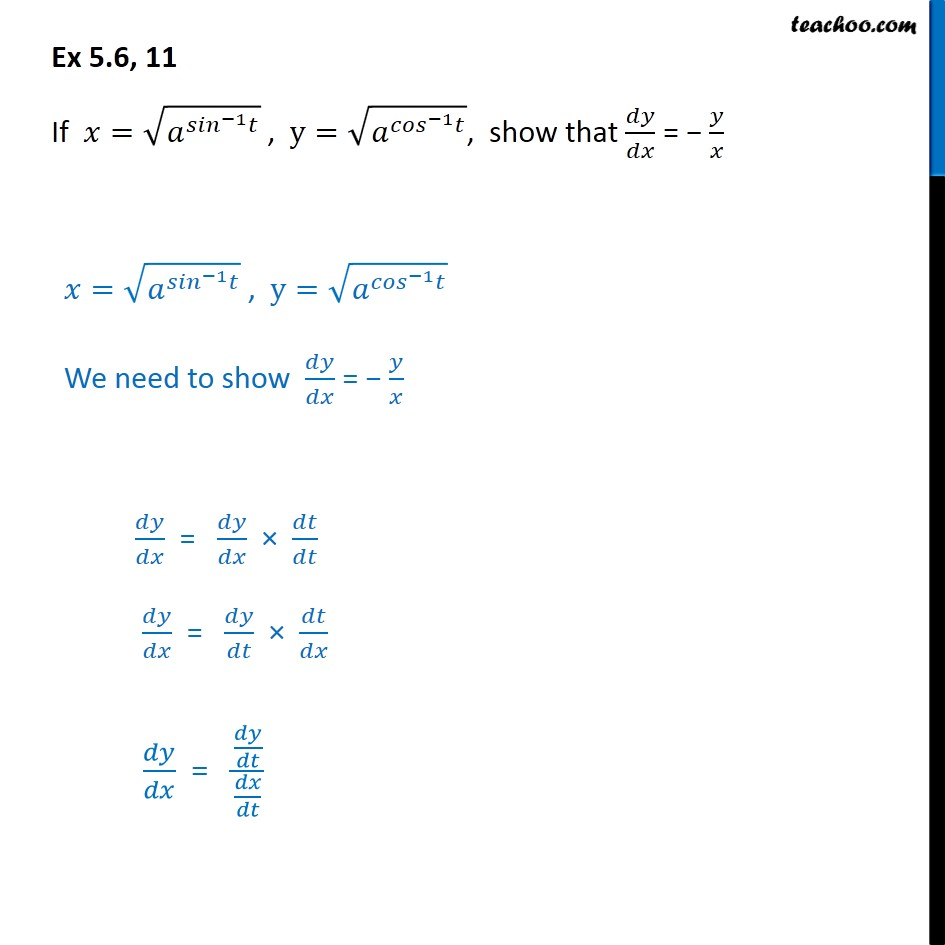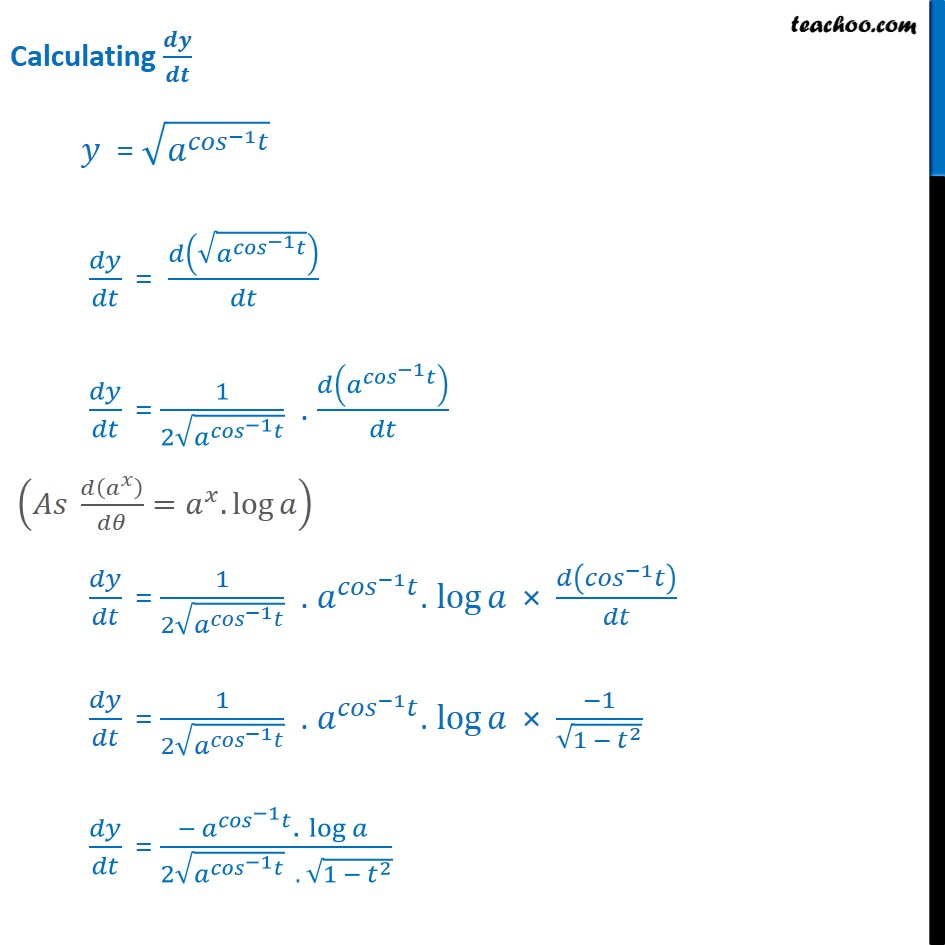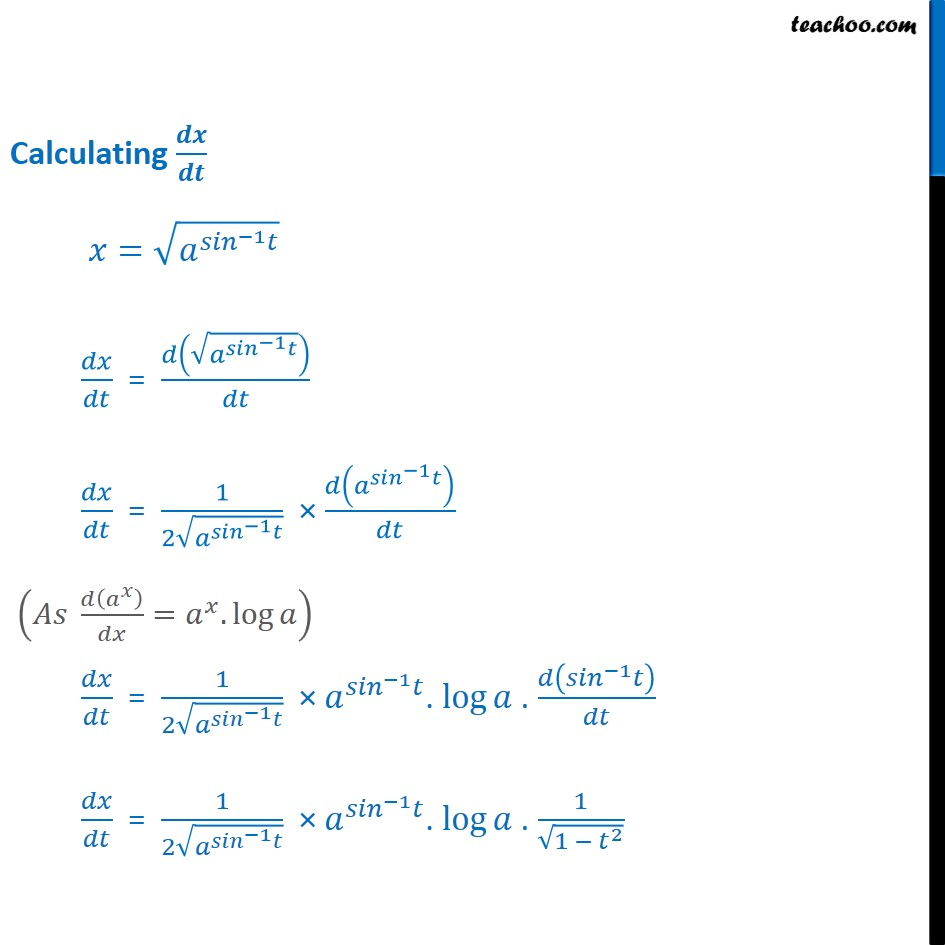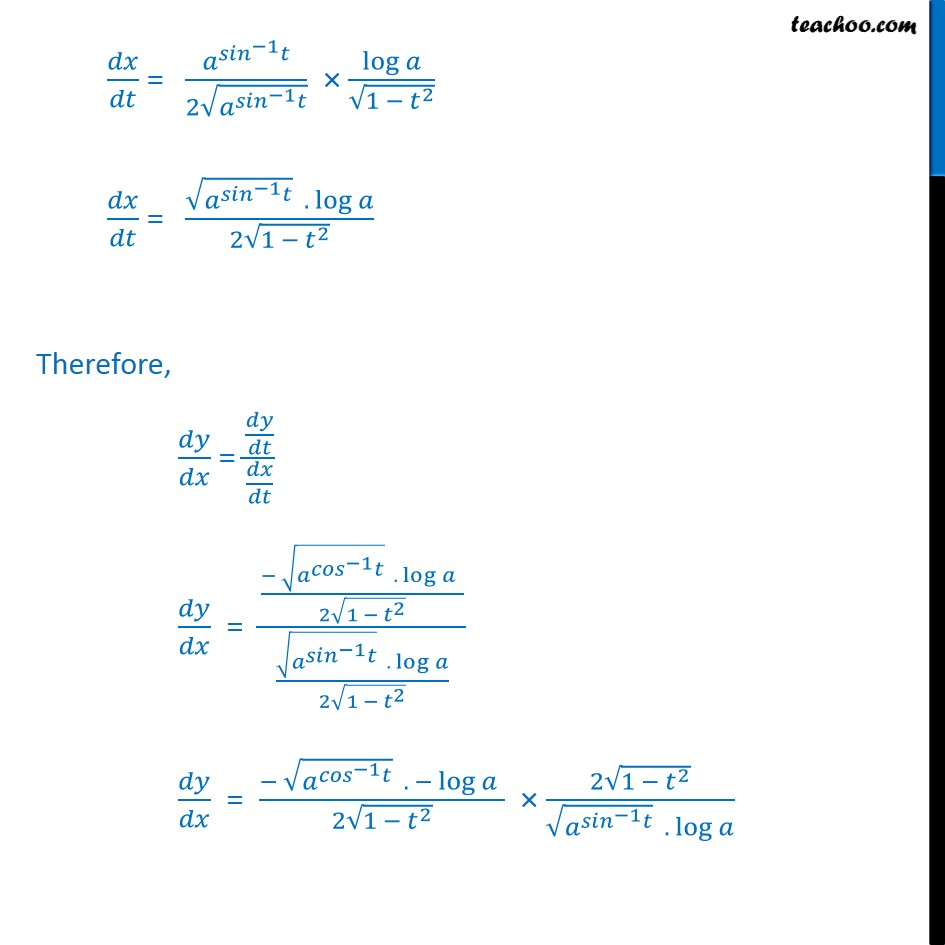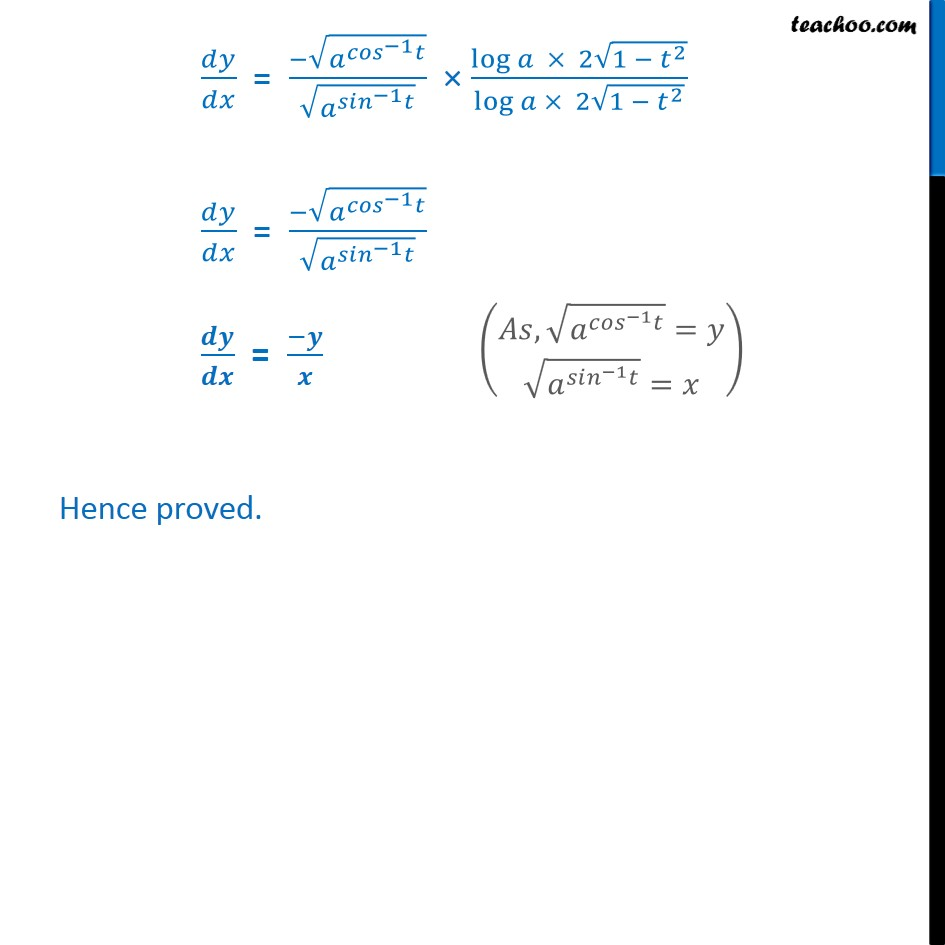1. Chapter 5 Class 12 Continuity and Differentiability
2. Serial order wise
3. Ex 5.6

Transcript

Ex 5.6, 11 If = 1 , y= 1 , show that = = 1 , y= 1 We need to show = = = = Calculating = 1 = 1 = 1 2 1 . 1 = 1 2 1 . 1 . log 1 = 1 2 1 . 1 . log 1 1 2 = 1 . log 2 1 . 1 2 Calculating = 1 = 1 = 1 2 1 1 = 1 2 1 1 . log . 1 = 1 2 1 1 . log . 1 1 2 = 1 2 1 log 1 2 = 1 . log 2 1 2 Therefore, = = 1 . log 2 1 2 1 . log 2 1 2 = 1 . log 2 1 2 2 1 2 1 . log = 1 1 log 2 1 2 log 2 1 2 = 1 1 = Hence proved.

Ex 5.6